# Comparison Operators in Excel

Use comparison operators in Excel to check if two values are equal to each other, if one value is greater than another value, if one value is less than another value, etc.

### Equal to

The equal to operator (=) returns TRUE if two values are equal to each other.

1. For example, take a look at the formula in cell C1 below.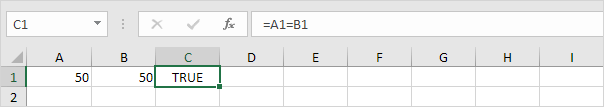Explanation: the formula returns TRUE because the value in cell A1 is equal to the value in cell B1. Always start a formula with an equal sign (=).

2. The IF function below uses the equal to operator.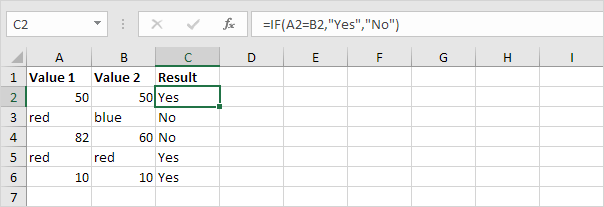Explanation: if the two values (numbers or text strings) are equal to each other, the IF function returns Yes, else it returns No.

### Greater than

The greater than operator (>) returns TRUE if the first value is greater than the second value.

1. For example, take a look at the formula in cell C1 below.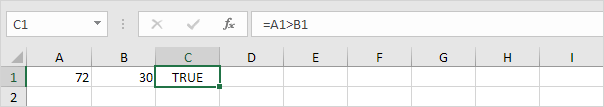Explanation: the formula returns TRUE because the value in cell A1 is greater than the value in cell B1.

2. The OR function below uses the greater than operator.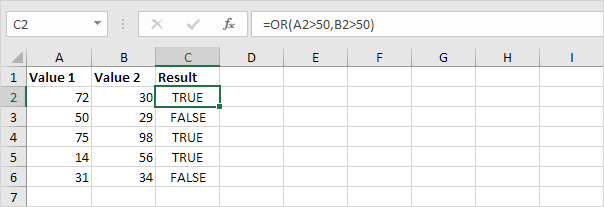Explanation: this OR function returns TRUE if at least one value is greater than 50, else it returns FALSE.

### Less than

The less than operator (<) returns TRUE if the first value is less than the second value.

1. For example, take a look at the formula in cell C1 below.Explanation: the formula returns TRUE because the value in cell A1 is less than the value in cell B1.

2. The AND function below uses the less than operator.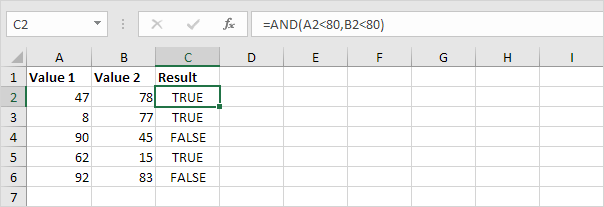Explanation: this AND function returns TRUE if both values are less than 80, else it returns FALSE.

### Greater than or equal to

The greater than or equal to operator (>=) returns TRUE if the first value is greater than or equal to the second value.

1. For example, take a look at the formula in cell C1 below.Explanation: the formula returns TRUE because the value in cell A1 is greater than or equal to the value in cell B1.

2. The COUNTIF function below uses the greater than or equal to operator.Explanation: this COUNTIF function counts the number of cells that are greater than or equal to 10.

### Less than or equal to

The less than or equal to operator (<=) returns TRUE if the first value is less than or equal to the second value.

1. For example, take a look at the formula in cell C1 below.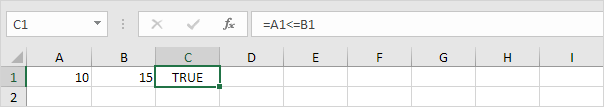Explanation: the formula returns TRUE because the value in cell A1 is less than or equal to the value in cell B1.

2. The SUMIF function below uses the less than or equal to operator.Explanation: this SUMIF function sums values in the range A1:A5 that are less than or equal to 10.

### Not Equal to

The not equal to operator (<>) returns TRUE if two values are not equal to each other.

1. For example, take a look at the formula in cell C1 below.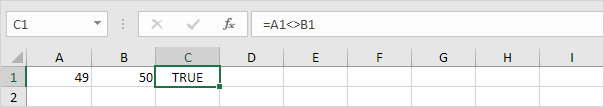Explanation: the formula returns TRUE because the value in cell A1 is not equal to the value in cell B1.

2. The IF function below uses the not equal to operator.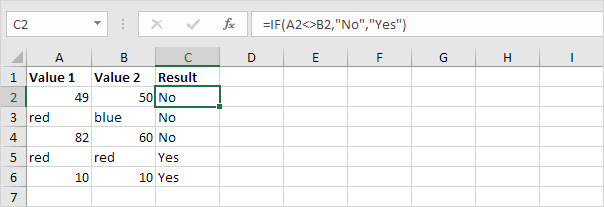Explanation: if the two values (numbers or text strings) are not equal to each other, the IF function returns No, else it returns Yes.

Go to Next Chapter: Cell References